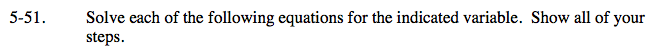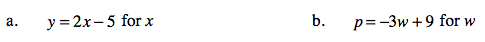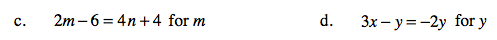### Home > CAAC > Chapter 5 > Lesson 5.1.5 > Problem5-51

5-51.Isolate the variable on one side.

y = 2x − 5
+5 + 5
y + 5= 2x

Solve for x.

$\frac{\textit{y} + 5}{2} = \textit{x}$

Follow the steps in part (a).Follow the steps in part (a).

m = 2n +5

Follow the steps in part (a).

y = −3x